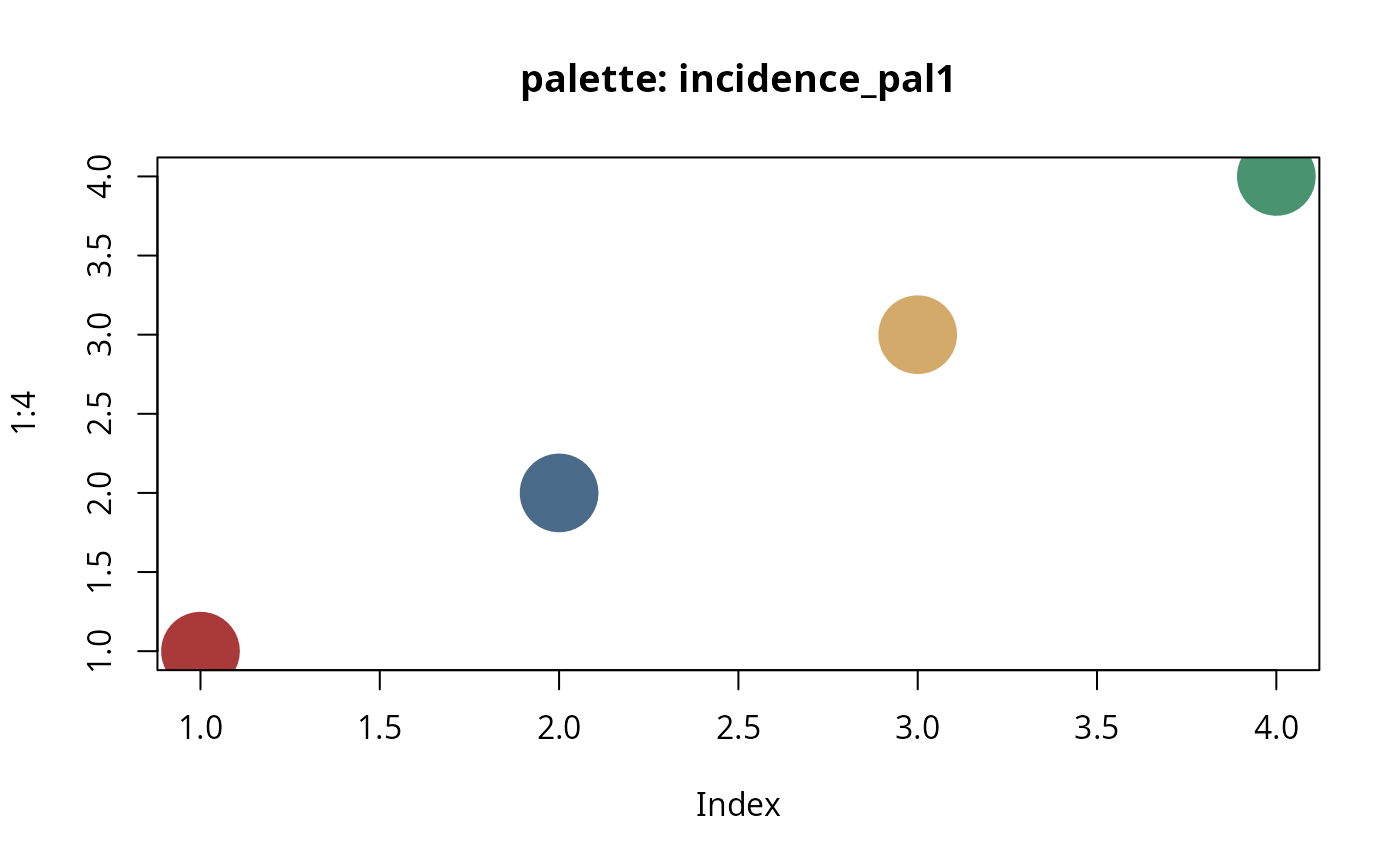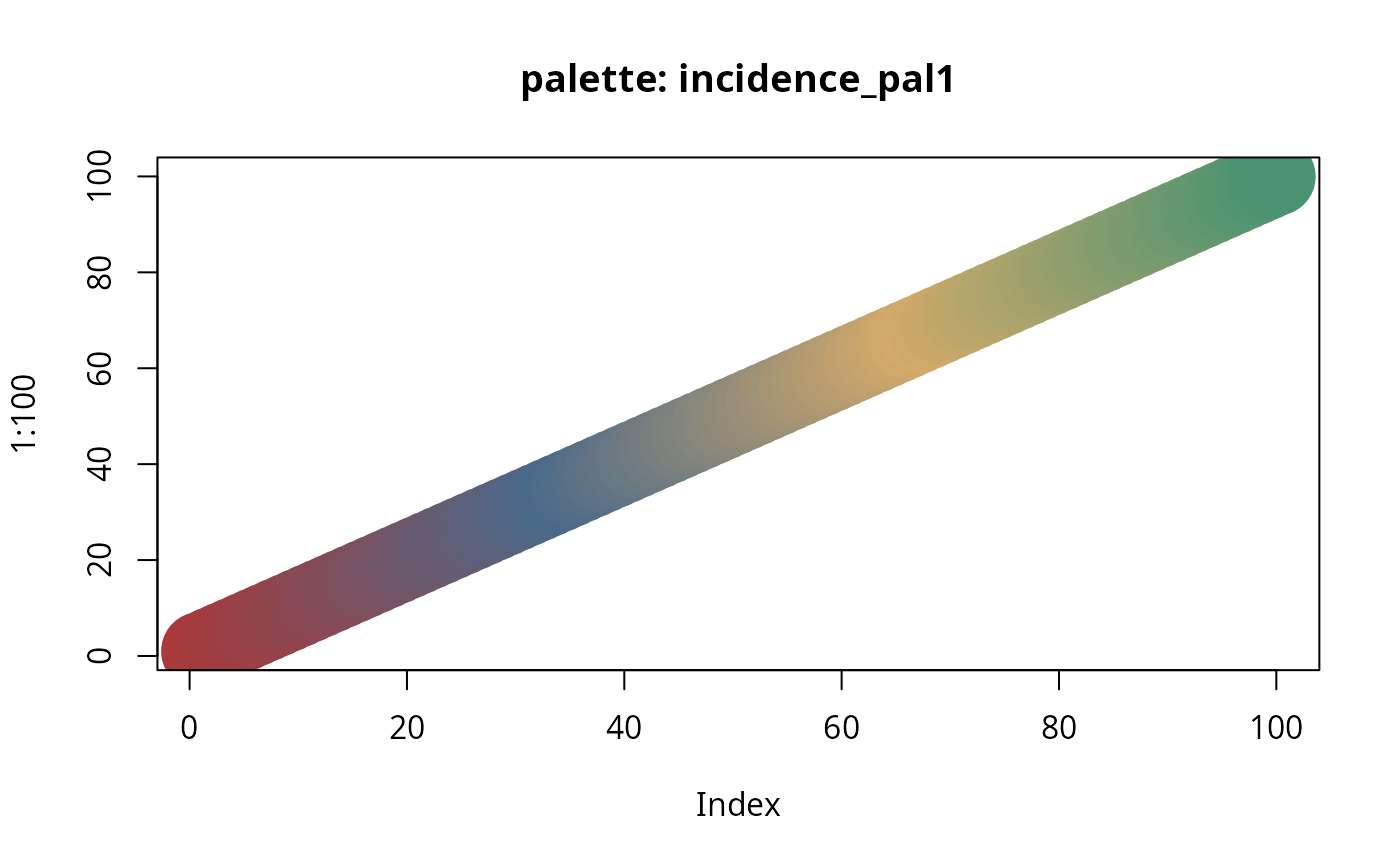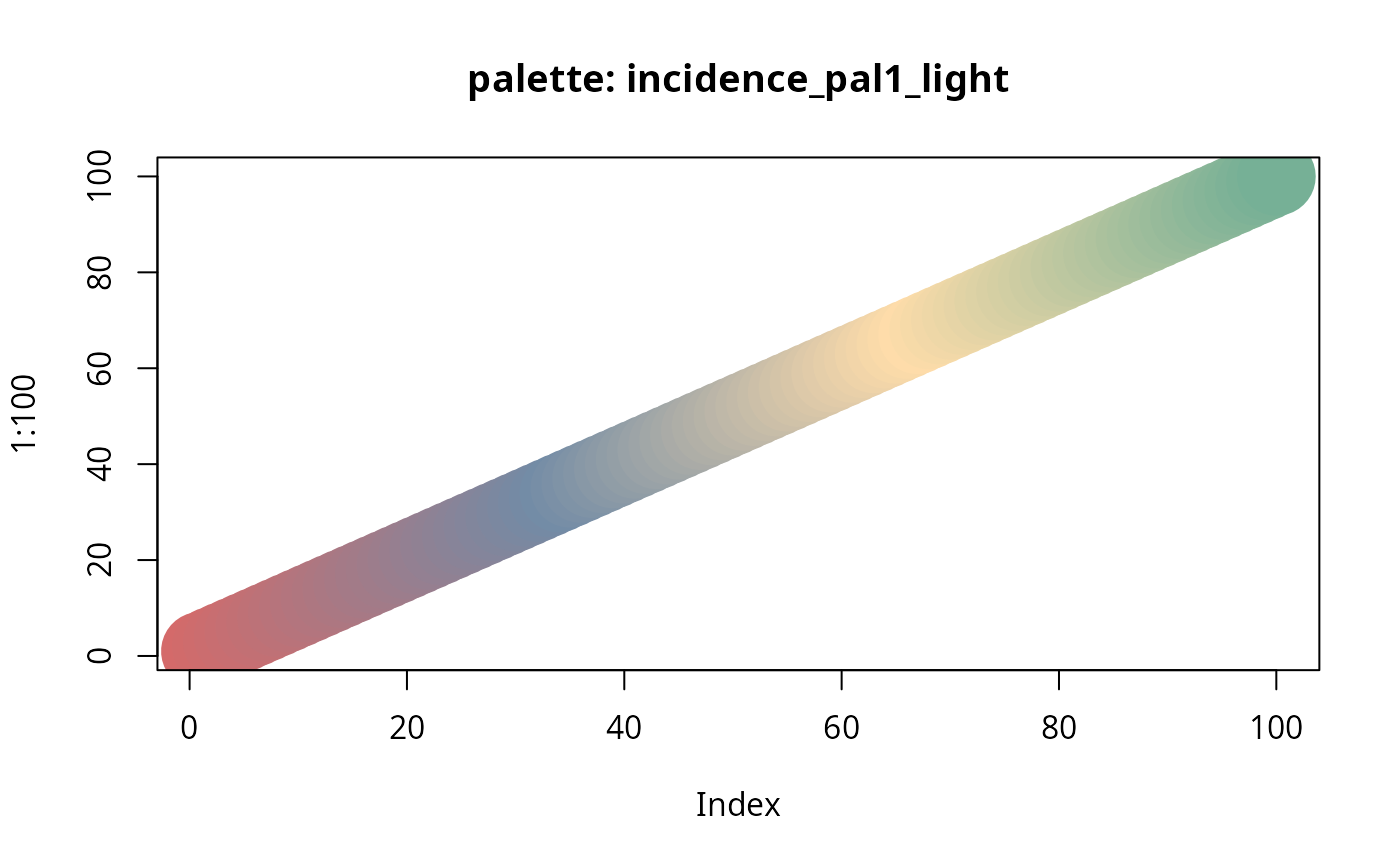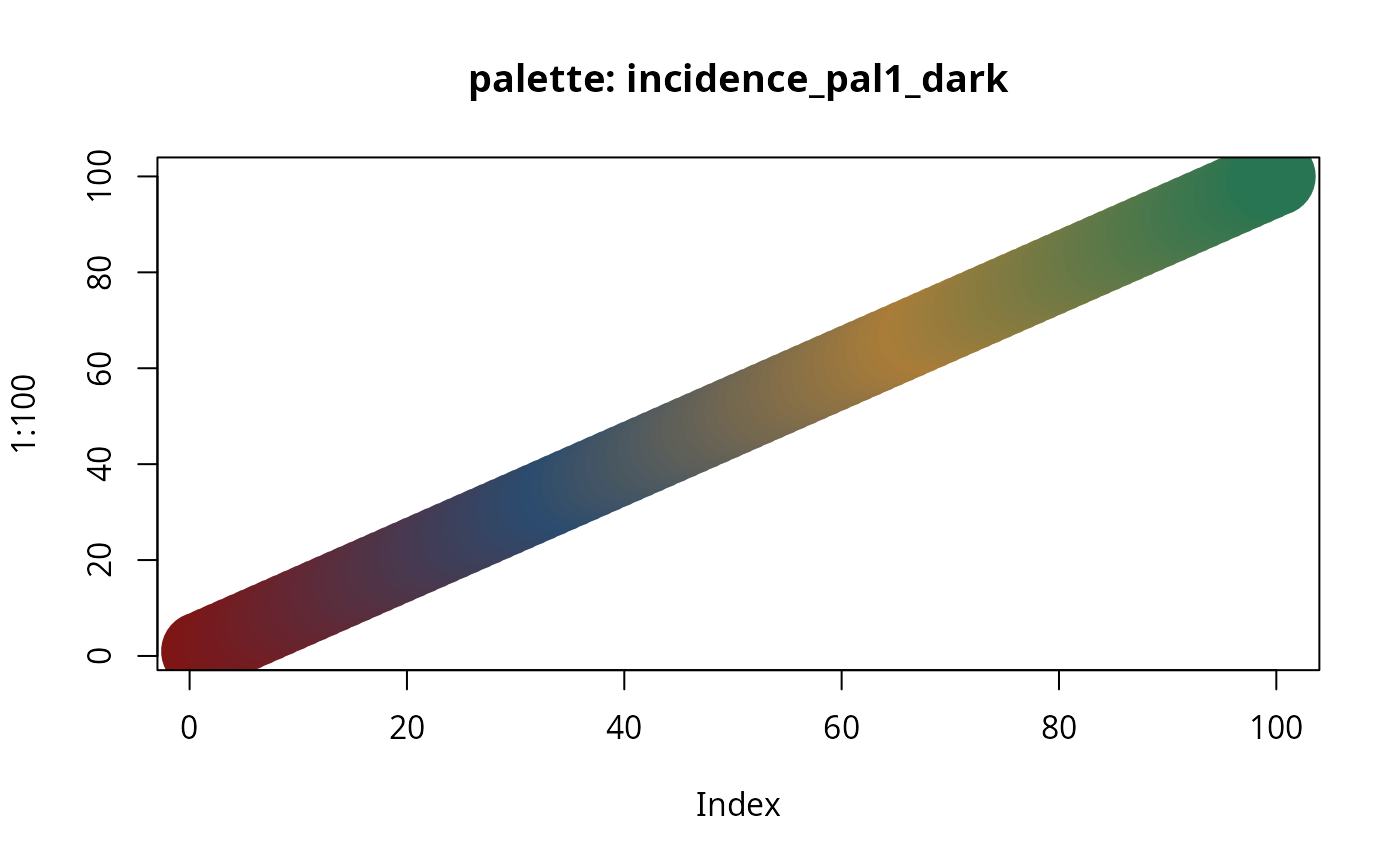These functions are color palettes used in incidence.

incidence_pal1(n)

incidence_pal1_light(n)

incidence_pal1_dark(n)

## Arguments

n a number of colors

## Examples


plot(1:4, cex=8, pch=20, col = incidence_pal1(4),
main = "palette: incidence_pal1")plot(1:100, cex=8, pch=20, col = incidence_pal1(100),
main ="palette: incidence_pal1")plot(1:100, cex=8, pch=20, col = incidence_pal1_light(100),
main="palette: incidence_pal1_light")plot(1:100, cex=8, pch=20, col = incidence_pal1_dark(100),
main="palette: incidence_pal1_dark")